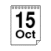#How to calculate the day of the week

## Twentieth century dates

For a date in the twentieth century, the method is slightly simpler than for other dates:

1. Take the year number (0 for 1900, 32 for 1932, 98 for 1998 etc.) and divide it by 4 (ignore any remainder)
2. Add the year number to the number calculated above
3. Add the date (day of the month) to the result
4. Add the month number from the table (right)
5. Divide by seven, and the remainder gives you the day of the week (starting with Monday as day 1):
1234560
MonTueWedThuFriSatSun

## For any Gregorian date

A similar method can be used for any day in any century AD (although remember that the Gregorian calendar was only invented in 1582!)

1. Take any year (including the century, eg. 1998 not 98)
2. Divide the year by 4 and ignore any remainder. Add this result onto the year
3. Divide the year by 100, ignore any remainder and subtract this from the total
4. Divide the year by 400, ignore any remainder and add this to the total
5. Add the date (day of the month)
6. Add the month number from the table as before
7. Subtract 1 from the total
8. This gives you the day of the week, as for the earlier method

### Example

```On which day of the week was the 9th of March 1879?
1879 / 4 = 469, 1879 + 469 = 2348
1879 / 100 = 18, 2348 - 18 = 2330
1879 / 400 = 4, 2330 + 4 = 2334
2334 + 9 = 2343
Using the month number from the table, 2343 + 3 = 2346
2346 - 1 = 2345
2345 / 7 = 335 remainder 0
So, 09/03/1879 was a Sunday
```

The only problem with the method described above is the need for a table of month numbers. If anyone knows a better method, I would much appreciate it if you would contact me using the button below.